Successfully reported this slideshow.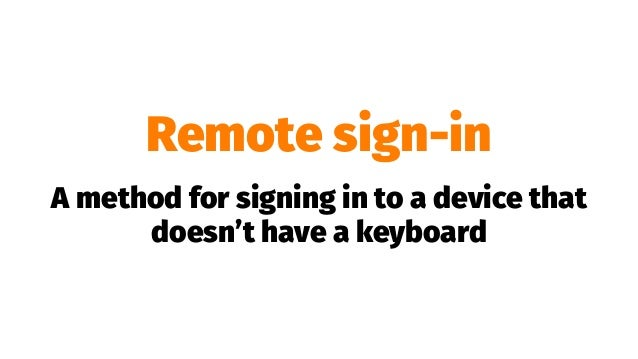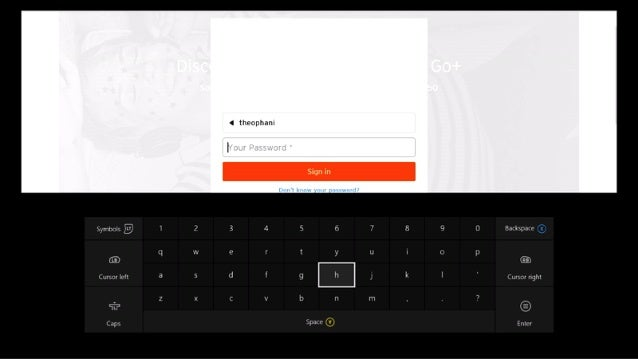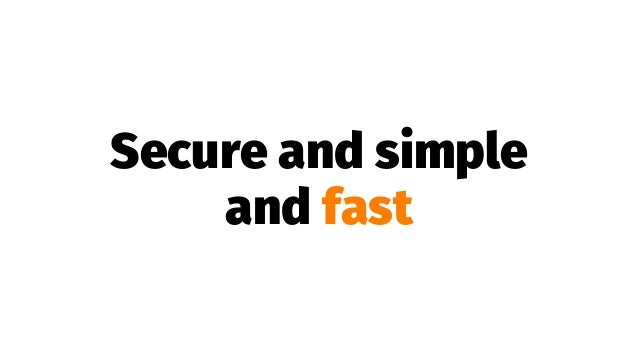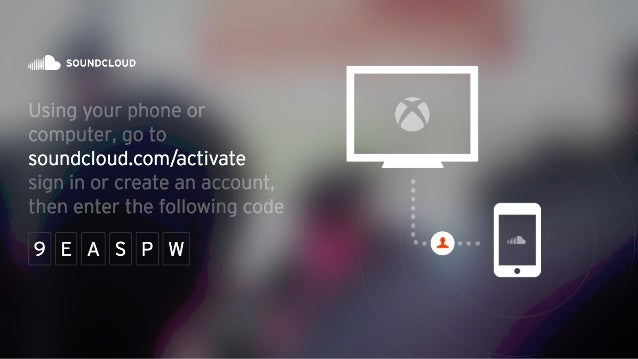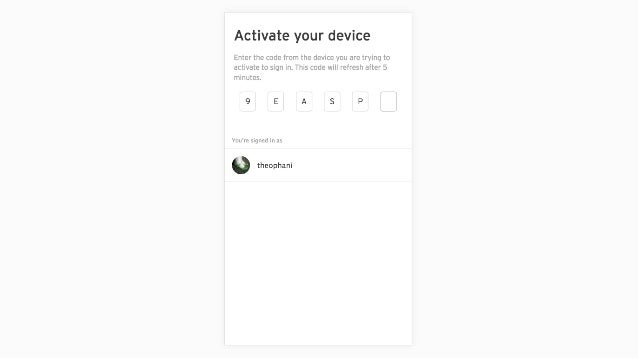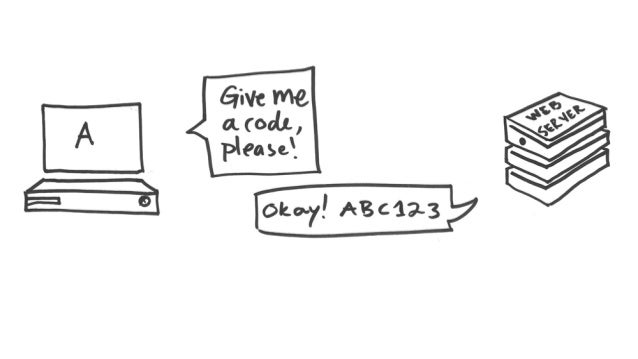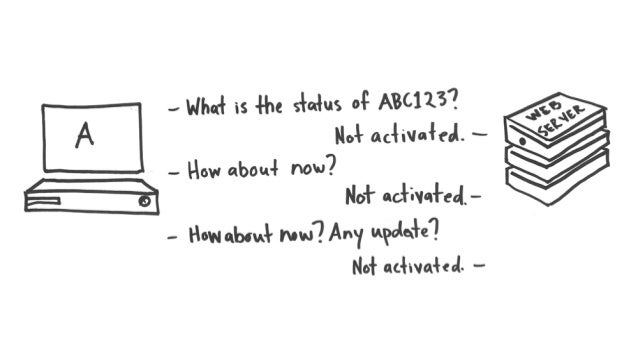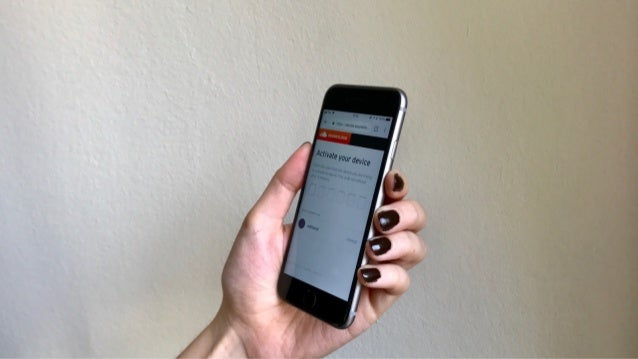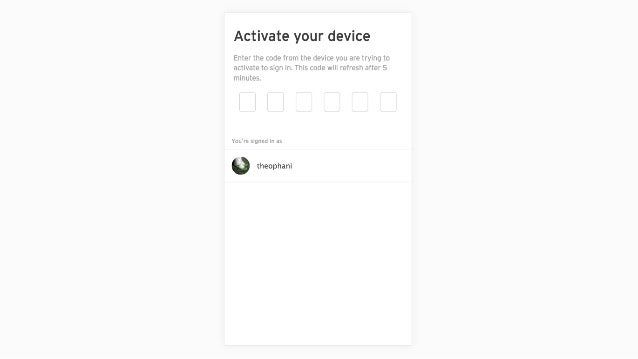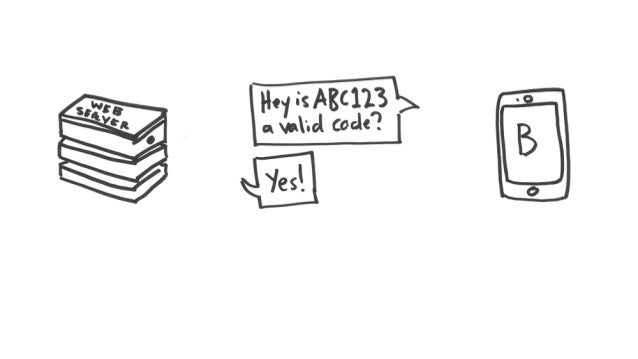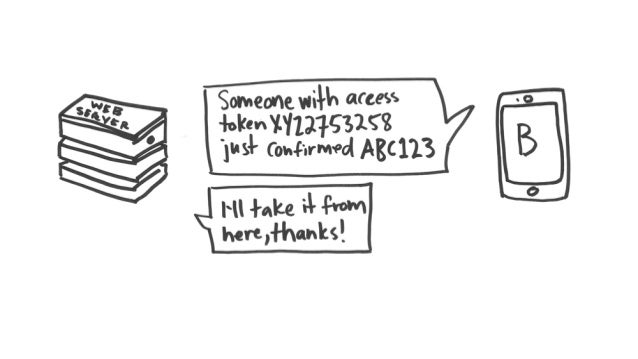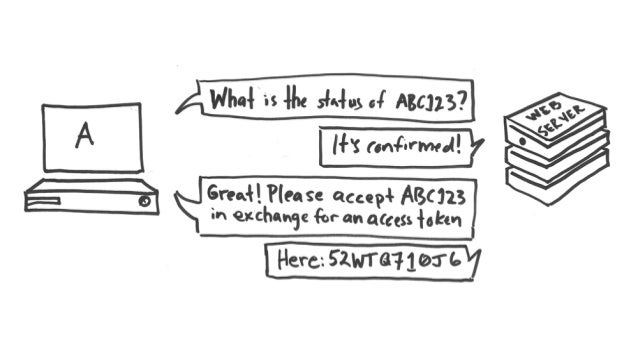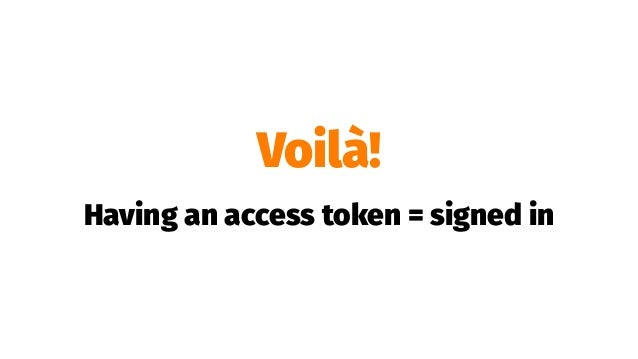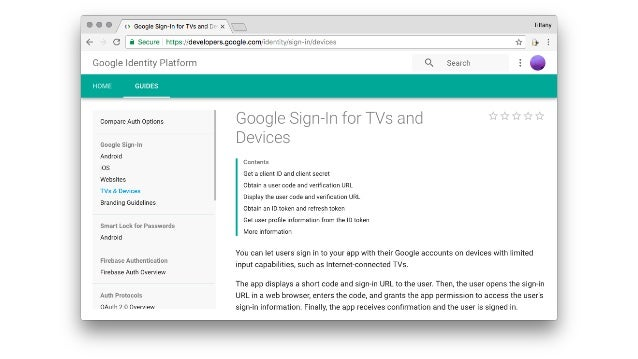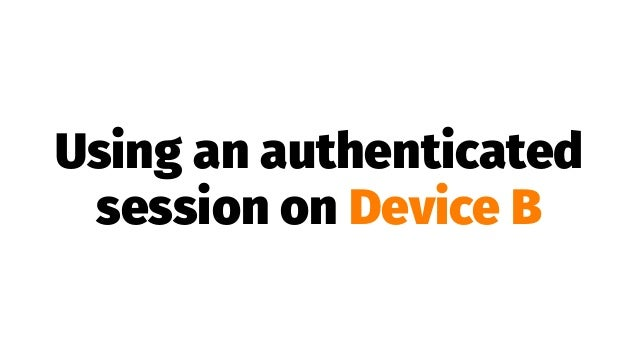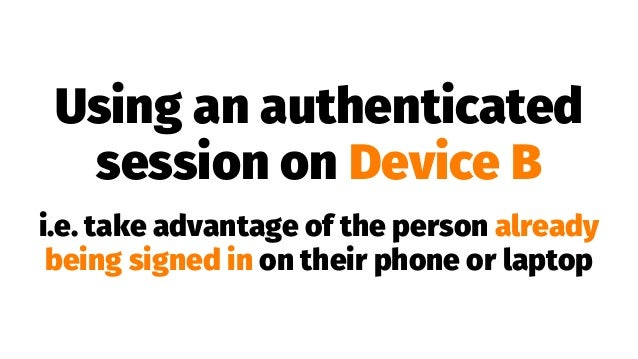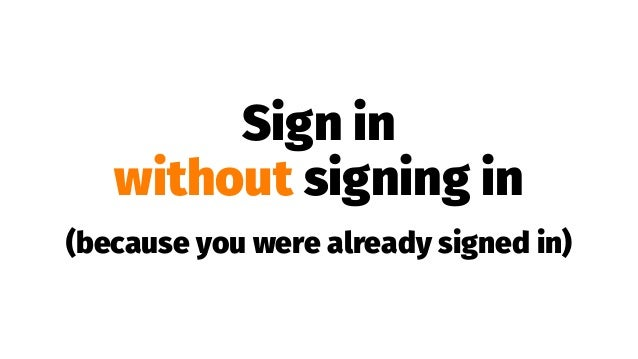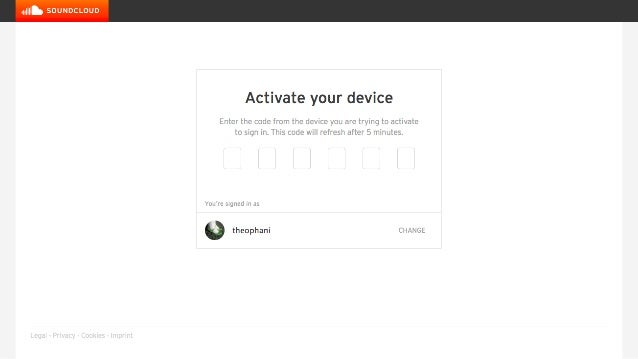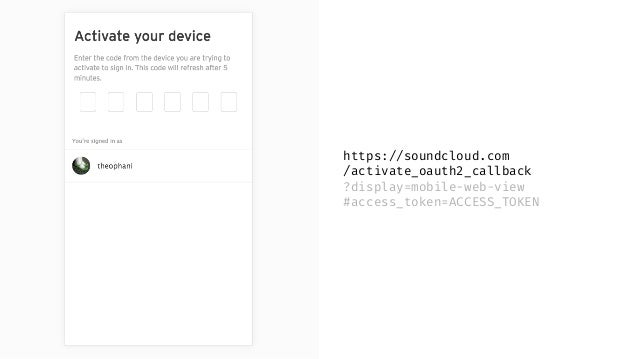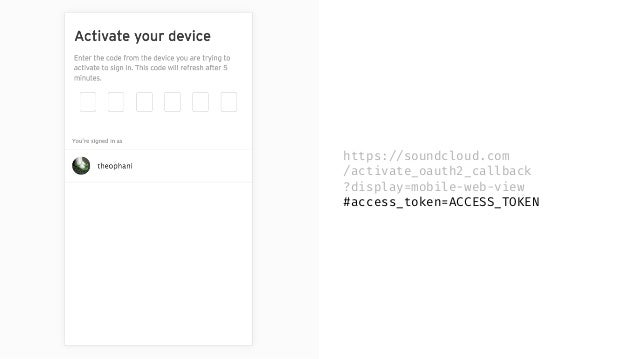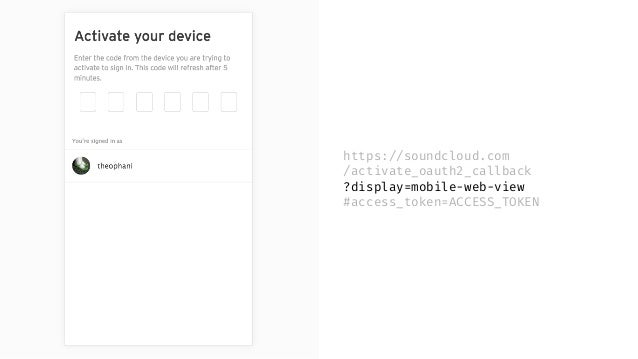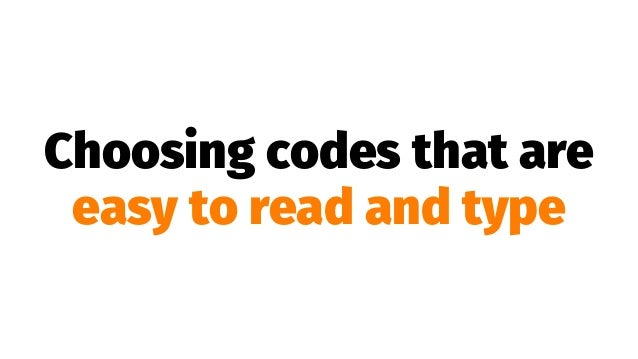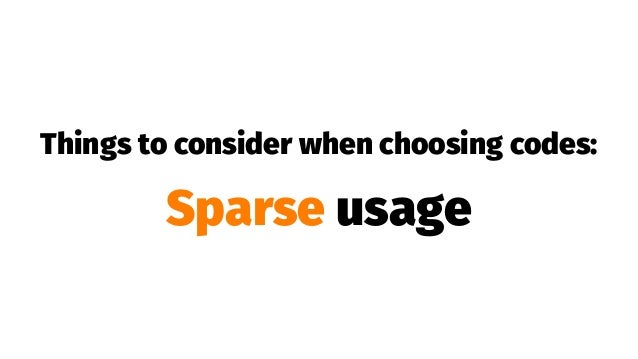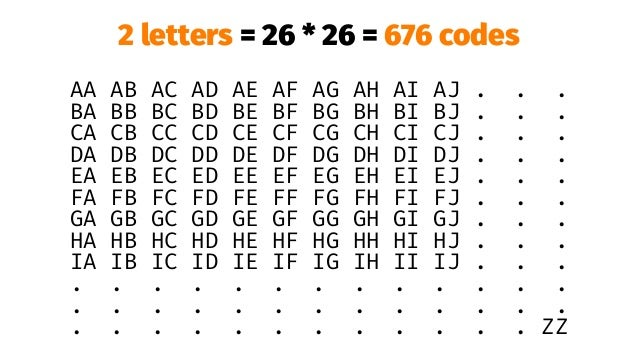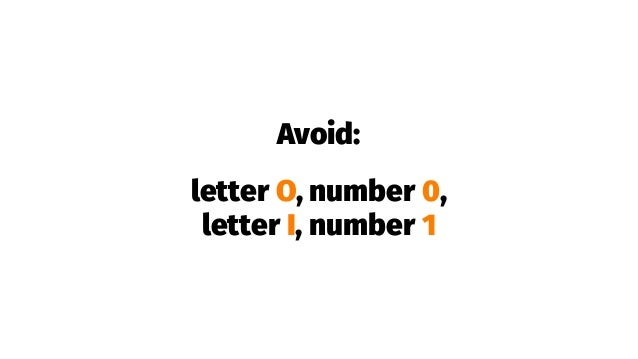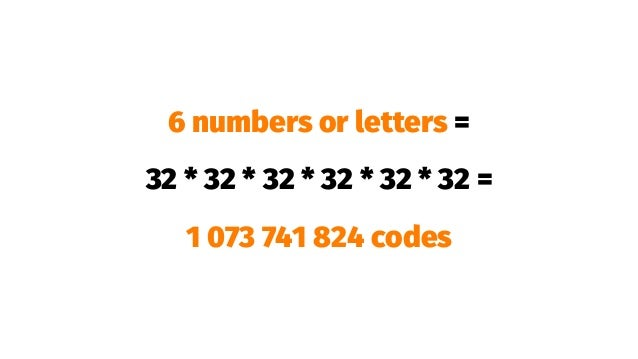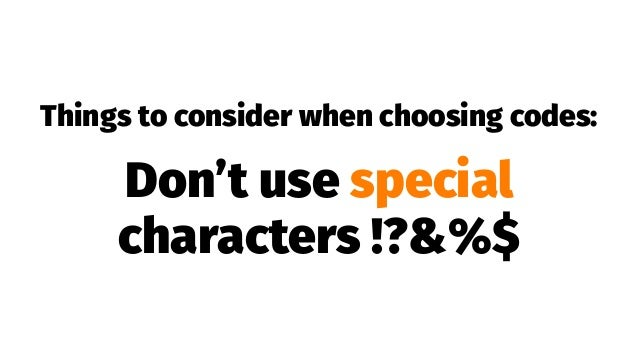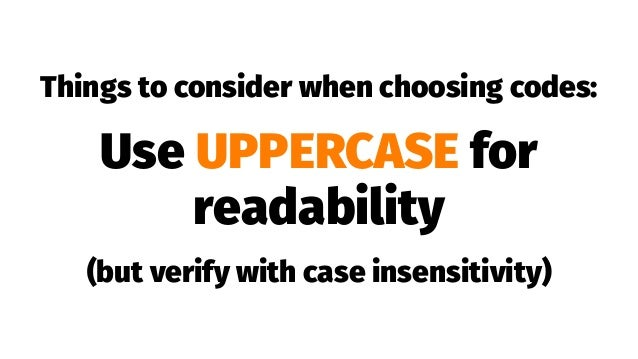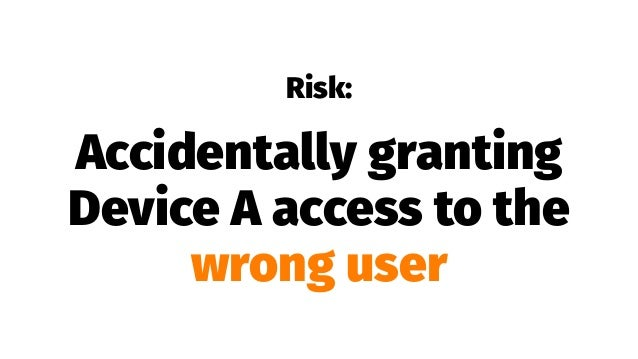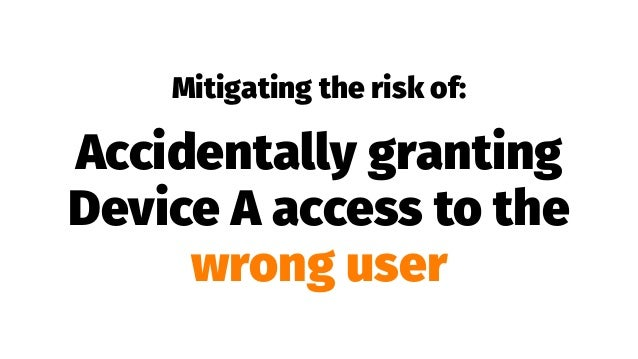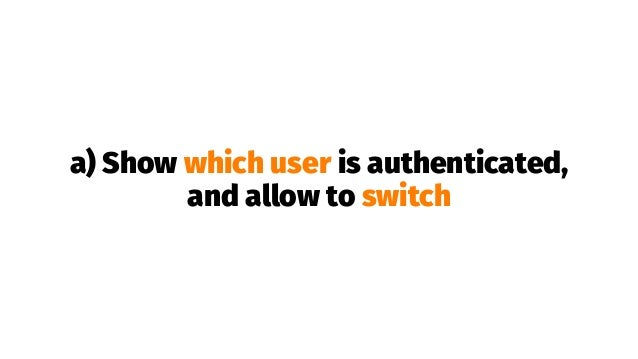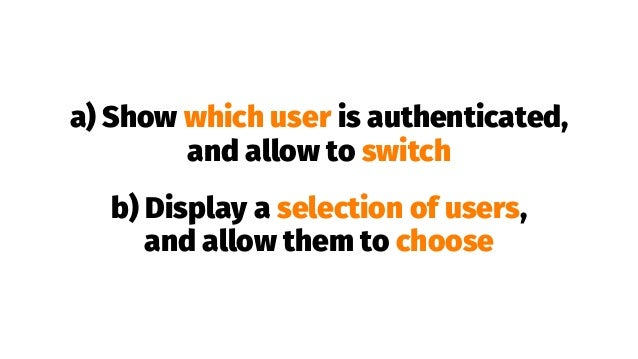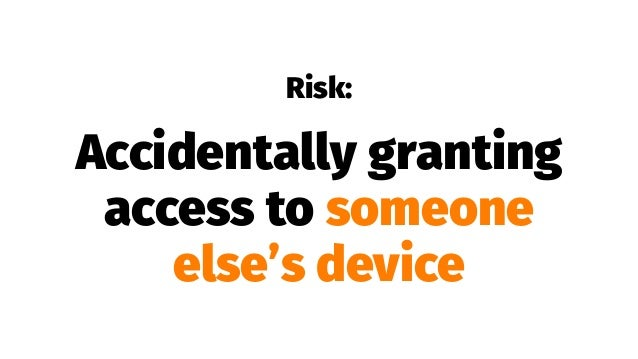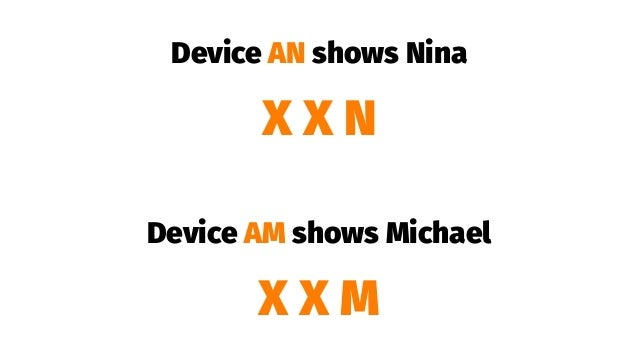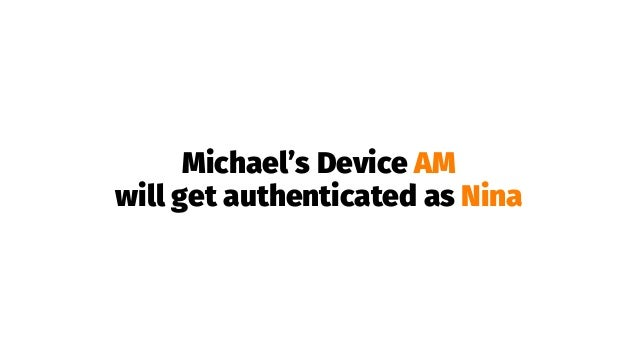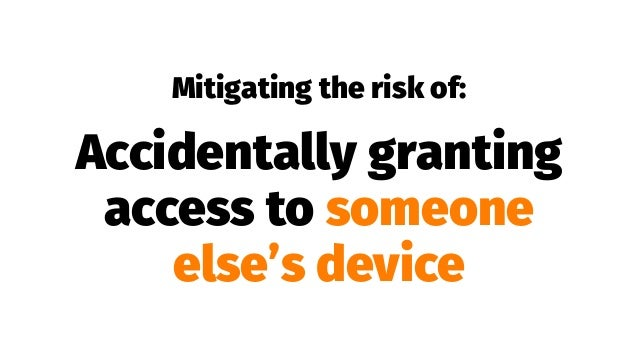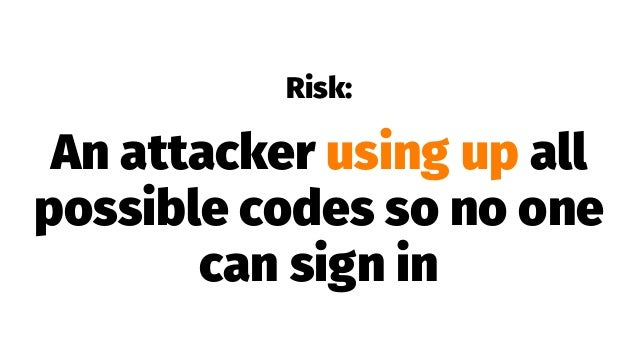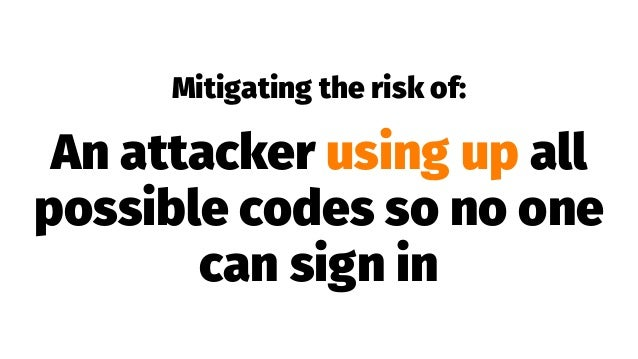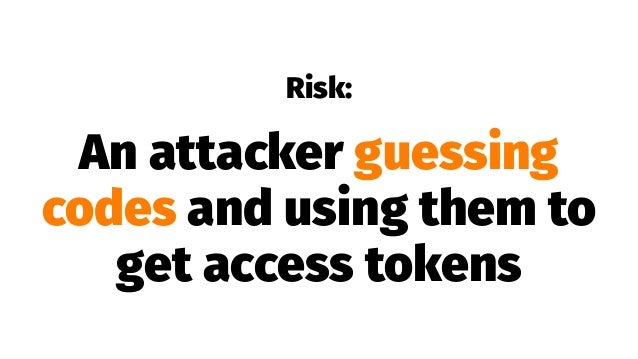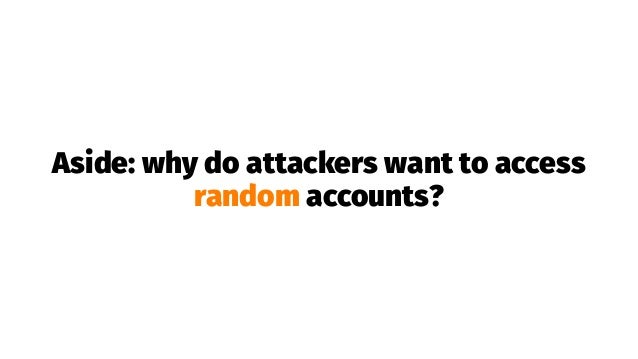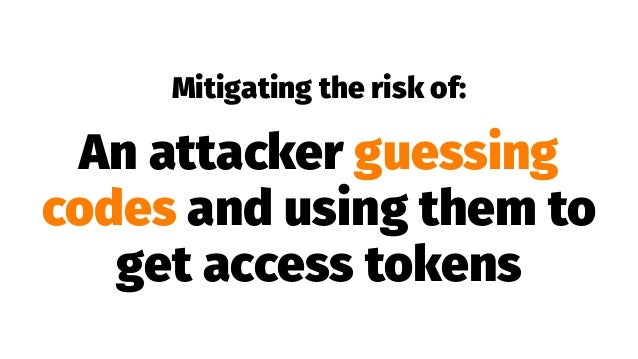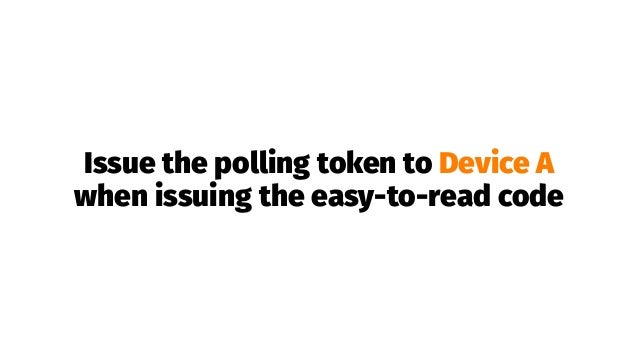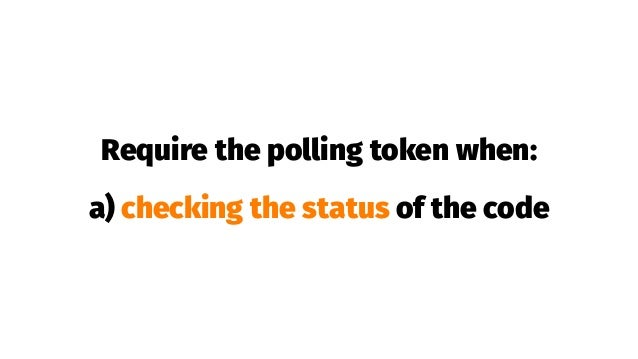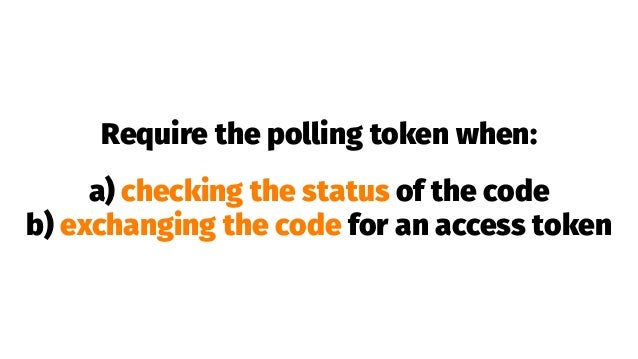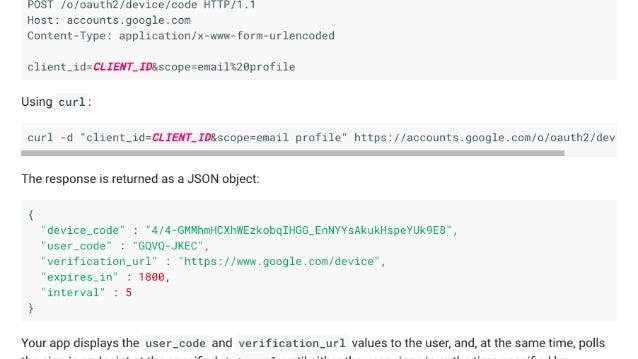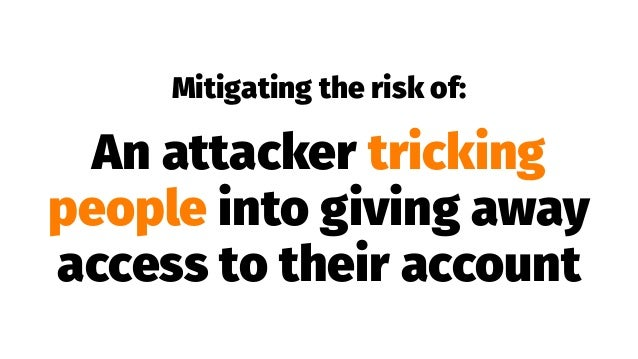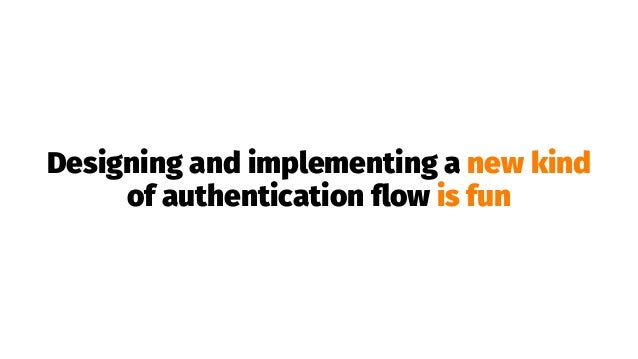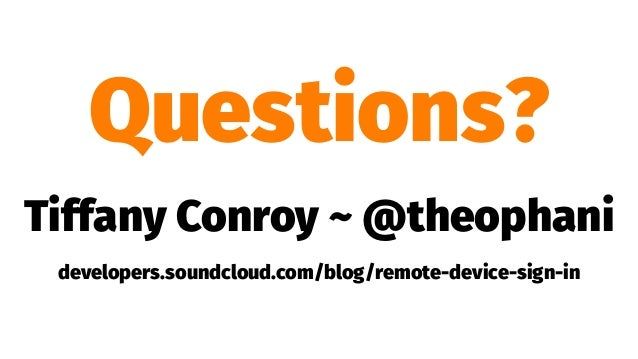Upcoming SlideShare
×
• Full Name
Comment goes here.

Are you sure you want to Yes No• Be the first to comment

• Be the first to like this

### Tiffany Conroy - Remote device sign-in – Authenticating without a keyboard - Codemotion Milan 2017

1. 1. Remote sign-in A method for signing in to a device that doesn’t have a keyboard
2. 2. Hi, I’m Tiffany @theophani
3. 3. Remote sign-in A method for signing in to a device that doesn’t have a keyboard
4. 4. SoundCloud on Xbox
5. 5. Signing in with a game controller is not fun
6. 6. Secure and simple and fast
7. 7. The solution, in brief
8. 8. How it works
10. 10. Inspiration: YouTube on TVs and Google Sign-in for TVs and Devices
11. 11. Using an authenticated session on Device B
12. 12. Using an authenticated session on Device B i.e. take advantage of the person already being signed in on their phone or laptop
15. 15. https://soundcloud.com /activate_oauth2_callback ?display=mobile-web-view #access_token=ACCESS_TOKEN
16. 16. https://soundcloud.com /activate_oauth2_callback ?display=mobile-web-view #access_token=ACCESS_TOKEN
17. 17. https://soundcloud.com /activate_oauth2_callback ?display=mobile-web-view #access_token=ACCESS_TOKEN
18. 18. Choosing codes that are easy to read and type
19. 19. Things to consider when choosing codes: Sparse usage
20. 20. . . . . . . . . . . . . . . . . . . . . . . . . . . . . . . . . . . . . . . . . . . . . . . . . . . . . . . . . . . . . X . . . . . . . . . . . . . . . . . . . . . . . . . . . . . . . . . . . . . . . . . . . . . . . . . . . . . . . . . . . . . . . . . . . . . . . . . . . . . . . . . . . . . . . . . . . . . . . . . . . . . . . . . . . . . . . . . . . . . . . . . . . . . . . . . . X . . . . . . . . . . . . . . . . . . . . . . . . . . . . . . . . . . . . . . . . . . . . . X . . . . . . . . . . . . . . . . . . . . . . . . . . . . . . . . . . . . . X . . . . . . . . . . X . . . . . . . . . . . . . . . . . . . . . . . . . . . . . . . . . . . . . . . . . . . . . . . . . . . . . . . . . . . . . . . . . . . . . . . . . . . . . . . . . . . . . . . . . . . . . . . X . . . . . . . . .
21. 21. 1 number = 10 codes 0 1 2 3 4 5 6 7 8 9
22. 22. 2 letters = 26 * 26 = 676 codes AA AB AC AD AE AF AG AH AI AJ . . . BA BB BC BD BE BF BG BH BI BJ . . . CA CB CC CD CE CF CG CH CI CJ . . . DA DB DC DD DE DF DG DH DI DJ . . . EA EB EC ED EE EF EG EH EI EJ . . . FA FB FC FD FE FF FG FH FI FJ . . . GA GB GC GD GE GF GG GH GI GJ . . . HA HB HC HD HE HF HG HH HI HJ . . . IA IB IC ID IE IF IG IH II IJ . . . . . . . . . . . . . . . . . . . . . . . . . . . . . . . . . . . . . . . . . ZZ
23. 23. 6 numbers = 1 000 000 codes 4 letters = 26 * 26 * 26 * 26 = 456 976 codes
24. 24. Numbers and letters?
25. 25. Avoid: letter O, number 0, letter I, number 1
26. 26. 6 numbers or letters = 32 * 32 * 32 * 32 * 32 * 32 = 1 073 741 824 codes
27. 27. Things to consider when choosing codes: Don’t use special characters !?&%\$
28. 28. Things to consider when choosing codes: Use UPPERCASE for readability (but verify with case insensitivity)
29. 29. Security considerations
30. 30. Risk: Accidentally granting Device A access to the wrong user
31. 31. Someone is signed in … but who?
32. 32. Mitigating the risk of: Accidentally granting Device A access to the wrong user
33. 33. a) Show which user is authenticated, and allow to switch
34. 34. a) Show which user is authenticated, and allow to switch b) Display a selection of users, and allow them to choose
36. 36. Device AN shows Nina X X N Device AM shows Michael X X M
37. 37. Nina accidentally types X X M
38. 38. Michael’s Device AM will get authenticated as Nina
39. 39. Mitigating the risk of: Accidentally granting access to someone else’s device
40. 40. Sparse usage of codes!
41. 41. . . . . . . . . . . . . . . . . . . . . . . . . . . . . . . . . . . . . . . . . . . . . . . . . . . . . . . . . . . . . X . . . . . . . . . . . . . . . . . . . . . . . . . . . . . . . . . . . . . . . . . . . . . . . . . . . . . . . . . . . . . . . . . . . . . . . . . . . . . . . . . . . . . . . . . . . . . . . . . . . . . . . . . . . . . . . . . . . . . . . . . . . . . . . . . . X . . . . . . . . . . . . . . . . . . . . . . . . . . . . . . . . . . . . . . . . . . . . ❌ X . . . . . . . . . . . . . . . . . . . . . . . . . . . . . . . . . . . . . X . . . . . . . . . . X . . . . . . . . . . . . . . . . . . . . . . . . . . . . . . . . . . . . . . . . . . . . . . . . . . . . . . . . . . . . . . . . . . . . . . . . . . . . . . . . . . . . . . . . . . . . . . . X . . . . . . . . .
42. 42. Collect device name to show during activation
43. 43. Risk: An attacker using up all possible codes so no one can sign in
44. 44. X X X X X X X X X X X X X X X X X X X X X X X X X X X X X X X X X X X X X X X X X X X X X X X X X X X X X X X X X X X X X X X X X X X X X X X X X X X X X X X X X X X X X X X X X X X X X X X X X X X X X X X X X X X X X X X X X X X X X X X X X X X X X X X X X X X X X X X X X X X X X X X X X X X X X X X X X X X X X X X X X X X X X X X X X X X X X X X X X X X X X X X X X X X X X X X X X X X X X X X X X X X X X X X X X X X X X X X X X X X X X X X X X X X X X X X X X X X X X X X X X X X X X X X X X X X X X X X X X X X X X X X X X X X X X X X X X X X X X X X X X X X X X X X X X X X X X X X X X X X X X X X X X X X X X X X X X X X X X X X X X X X X X X X X X X X X X X X X X X X X X X X X X X X X X X X X X X X X X X X X X X X X X X X X X X X X X X X X X X X X X X X X X X X X X X X
45. 45. Mitigating the risk of: An attacker using up all possible codes so no one can sign in
46. 46. Rate limit ability to request codes
47. 47. Expire codes
48. 48. Expire codes … but don’t reuse too soon
49. 49. Risk: An attacker guessing codes and using them to get access tokens
50. 50. Brute force attack
51. 51. Aside: why do attackers want to access random accounts?
52. 52. Mitigating the risk of: An attacker guessing codes and using them to get access tokens
53. 53. Very, VERY, sparse code usage?
54. 54. Rate limit for polling?
55. 55. Polling tokens
56. 56. e.g. AHDNFDJR-937JJ5N7HN-SNVKDHKSM2- FJSNMNDFF-93HF7H46AGMS
57. 57. Issue the polling token to Device A when issuing the easy-to-read code
58. 58. Require the polling token when: a) checking the status of the code
59. 59. Require the polling token when: a) checking the status of the code b) exchanging the code for an access token
60. 60. Risk: An attacker tricking people into giving away access to their account
61. 61. Social engineering attack
62. 62. Mitigating the risk of: An attacker tricking people into giving away access to their account
63. 63. Use text and design elements that make it clear
64. 64. Have short expirations
65. 65. Closing thoughts
66. 66. Using a game controller to enter a password is not fun
67. 67. Designing and implementing a new kind of authentication ﬂow is fun
68. 68. Involve your security experts early
69. 69. Painful → Magical
70. 70. Thanks :)
71. 71. Questions? Tiffany Conroy ~ @theophani developers.soundcloud.com/blog/remote-device-sign-in

Total views

412

On Slideshare

0

From embeds

0

Number of embeds

1

6

Shares

0